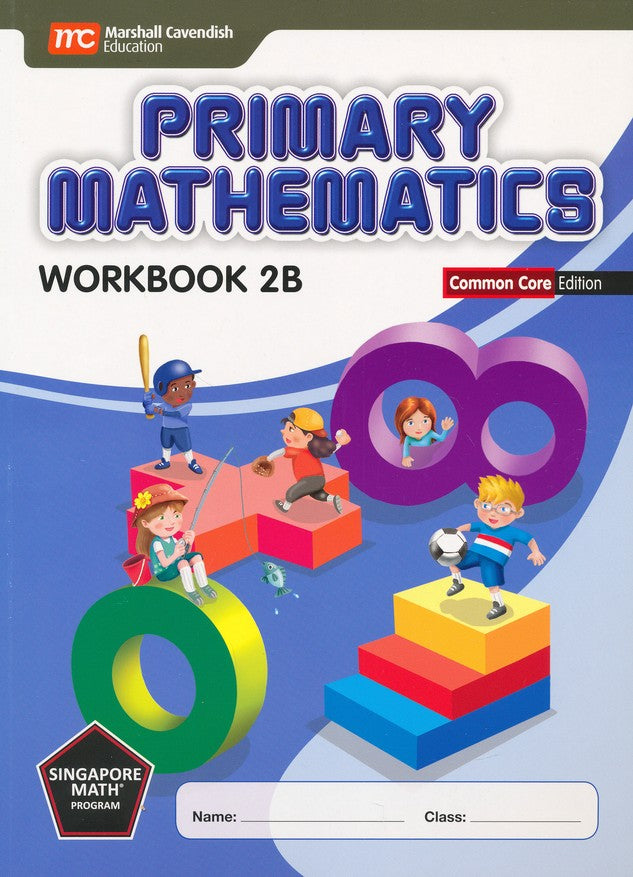# Singapore Math: Primary Math Workbook 2B Common Core Edition

\$ 17.50

Singapore Math Primary Mathematics: Common Core Edition books have been updated where necessary to align with the CCSS. However, this does not mean that the content has been reduced in its level of difficulty. If CCSS called for content to be introduced at a later level, Singapore Math didn't move content from the earlier level, but rather simply revisits it more in-depth at the CCSS level.

Singapore Math's Primary Mathematics workbooks are consumable and should be used in conjunction with the corresponding textbooks; these Common Core Edition workbooks are not compatible with other Singapore editions. Containing independent student exercises, workbooks provide the practice essential to skill mastery; these workbook sheets correspond to each chapter in the (sold-separately) textbook.

A variety of exercises are presented in the concrete>pictorial>abstract approach, including teaching children addition and subtraction, multiplication and division, money, fractions, time, tables and graphs, and geometry. 174 pages, softcover. Grade 2. Workbook 2B is designed for the 2nd semester of the Grade 2 year.

This workbook accompanies the sold-separately Primary Mathematics Common Core Edition Textbook 2B. Answer keys are sold separately here.

ISBN-13: 9789810198442